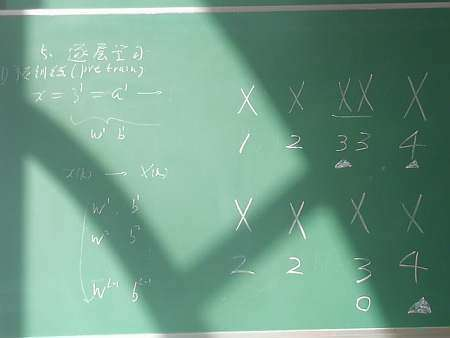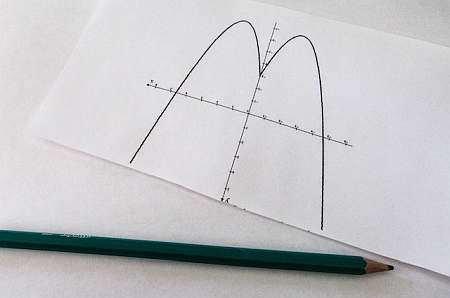# Polynomial Calculator

Instructions: Use this polynomial calculator to compute and simplify any polynomial operation that you provide, showing all the steps. Please type in polynomial expression you want to simplify in the the form box below.Enter the polynomial operation you want to calculate and/or simplify (Ex: f(x) = (x^2+2x)*x - 2/3x + x^2 + 4/5, etc.)

## Polynomial Calculator

This calculator will allow you to conduct polynomials calculations and simplifications, of a polynomial expression you provide, such as 3x^2 - 2/3 x + 1/4 + 5/4 - 3/4 x^2, etc.

You can also provide a more complicated polynomial expressions like 2/3 x^2(x - 3/4) + 5/4, provided that the result is a valid polynomial expression.

Once a valid polynomial is given, you can click on "Calculate" and the results of the calculation and simplification will be shown to you , showing all the steps of the process.

The calculations will be carried out using the usual PEMDAS criteria, for the priority and the order of operations.## How to calculate polynomials?

Despite the fact that polynomials can look scary, they are pretty amenable towards easy calculations, considering their linear nature. A general polynomial of degree $$n$$ has the following formula

$f(x) = a_0 + a_1 x + a_2 x^2 + a_3 x^3 + ... + a_n x^n$

## What are the steps for doing a polynomial calculation?

• Step 1: Identify the polynomial expression you need to calculate and simplify
• Step 2: Do a consistency check to find clear signs that the function is not polynomial. If that is the case, then you stop
• Step 3: Expand and simplify the terms inside of the polynomial expression by following the PEMDAS rule
• Step 4: Expand and simplify until no more simplifications can be conducted

Observe that polynomials have really neat closure properties. This is, if you add or subtract polynomials, you also get a polynomial. Also, if you multiply polynomials, the output is a polynomial as well. This is not necessarily true for division of polynomials.## Polynomial Division

Division is one operation without the closure property. This is, if you divide two polynomials, the outcome does not have to necessarily be a polynomial. It could be a polynomial, but it does not necessarily have to be one.

For example, you divide the polynomial $$f(x) = x^3 + 9x^2 + 27x +27$$ by the polynomial $$g(x) = x + 3$$, then the outcome is another polynomial:

$\displaystyle \frac{f(x)}{g(x)} = \displaystyle \frac{x^3 + 9x^2 + 27x +27}{x + 3} = x^2 + 6x + 9$

But then, if you divide the polynomial $$f(x) = x^3 + 9x^2 + 27x +28$$ by the polynomial $$g(x) = x + 3$$, then the outcome is NOT a polynomial.

## Why are polynomials important?

Polynomials are a very natural object that appears in applications. For example, quadratic equations are polynomials of order (degree) 2. So, it is only natural to work with polynomials of degree higher than 2.

It is true that quadratic functions take a much more center role in applications in basic Algebra, but that does not mean that higher degree polynomials do not have a preponderant place.### Example: Calculating polynomials

Expand and simplify the following: $$f(x) = 3x^2 - \frac{2}{3} x + \frac{1}{4} + \frac{5}{4} - \frac{3}{4} x^2$$

Solution:We are provided with the following expression: $$\displaystyle 3x^2 - \frac{2}{3} x + \frac{1}{4} + \frac{5}{4} - \frac{3}{4} x^2$$.

The following calculation is obtained:

$$\displaystyle 3x^2-\frac{2}{3}x+\frac{1}{4}+\frac{5}{4}-\frac{3}{4}x^2$$
Putting together the terms with $$x^2$$
$$\displaystyle = \,\,$$
$$\displaystyle -\frac{2}{3}x+\left(3-\frac{3}{4}\right)x^2+\frac{1}{4}+\frac{5}{4}$$
Putting the fractions together and simplifying the terms that were grouped with $$x^2$$
$$\displaystyle = \,\,$$
$$\displaystyle -\frac{2}{3}x+\frac{9}{4}x^2+\frac{1}{4}+\frac{5}{4}$$
Grouping and operating all the integer terms and fractions: $$\displaystyle \frac{ 1}{ 4}+\frac{ 5}{ 4}=\frac{ 1+5}{ 4}=\frac{ 6}{ 4}=\frac{ 2 \times 3}{ 2 \times 2}=\frac{ \cancel{ 2} \times 3}{ \cancel{ 2} \times 2}=\frac{ 3}{ 2}$$
$$\displaystyle = \,\,$$
$$\displaystyle -\frac{2}{3}x+\frac{9}{4}x^2+\frac{3}{2}$$
Reorganizing/simplifying/expanding the expression
$$\displaystyle = \,\,$$
$$\displaystyle \frac{9}{4}x^2-\frac{2}{3}x+\frac{3}{2}$$

which concludes the process of simplification.

### Example: Polynomial Calculator example

Calculate the following: $$f(x) = \frac{1}{3} x \left( \frac{5}{4}x - \frac{5}{6}\right)+x$$

Solution: Now we have the polynomial expression: $$\displaystyle \frac{1}{3}x\left(\frac{5}{4}x-\frac{5}{6}\right)+x$$.

The following simplification is obtained:

$$\displaystyle \frac{1}{3}x\left(\frac{5}{4}x-\frac{5}{6}\right)+x$$
Observe that $$(\frac{1}{3}x) \cdot (\frac{5}{4}x-\frac{5}{6}) = \frac{1}{3}x\cdot\frac{5}{4}x-\frac{5}{6}\cdot\frac{1}{3}x = \frac{5}{12}x^2-\frac{5}{18}x$$, by using the distributive property on each term of the expression on the left, with respect to the terms on the right
$$\displaystyle = \,\,$$
$$\displaystyle \frac{5}{12}x^2-\frac{5}{18}x+x$$
Aggregating those terms with $$x$$
$$\displaystyle = \,\,$$
$$\displaystyle \left(- \frac{ 5}{ 18}+1\right)x+\frac{5}{12}x^2$$
Operating the terms that were grouped with $$x$$
$$\displaystyle = \,\,$$
$$\displaystyle \frac{13}{18}x+\frac{5}{12}x^2$$

And that folks, is how you turn a hot mess into a semi-hot mess! The end of simplification has been reached.

### Example: Another Polynomial Calculator example

Expand and simplify $$f(x) = \left(\frac{2}{3}x - \frac{6}{5} \right)+ \frac{2}{5}x + 3$$.

Solution: We now have $$\displaystyle \left(\frac{2}{3}x-\frac{6}{5}\right)+\frac{2}{5}x+3$$.

We want to simplify this:

$$\displaystyle \frac{2}{3}x-\frac{6}{5}+\frac{2}{5}x+3$$
Grouping the terms with $$x$$
$$= \,\,$$
$$\displaystyle \left(\frac{2}{3}+\frac{2}{5}\right)x+3-\frac{6}{5}$$
Grouping together numerical values and fractions and simplifying the terms that were grouped with $$x$$
$$= \,\,$$
$$\displaystyle \frac{16}{15}x+3-\frac{6}{5}$$
Grouping and operating all the integer terms and fractions: $$\displaystyle 3-\frac{ 6}{ 5}=3 \times \frac{ 5}{ 5}-\frac{ 6}{ 5}=\frac{ 3 \times 5-6}{ 5}=\frac{ 15-6}{ 5}=\frac{ 9}{ 5}$$
$$= \,\,$$
$$\displaystyle \frac{16}{15}x+\frac{9}{5}$$

which ends the calculation.

## More algebra calculators

Polynomials are present in so many applications, and are one of the most important basic functions in Algebra. One of the particular case of polynomials is the case of quadratic functions, that are one of the simplest polynomials we will ever find.

You can do a lot of thing with them: you can graph polynomials, find its roots, look for symmetries and all that, but the easiest interpretation of all that happen for quadratic equations.